Next: Dispersive Waves Up: Wave Pulses Previous: Bandwidth

# Exercises

1. Verify Equations (701)-(703). Derive Equations (704) and (705) from Equation (699) and Equations (701)-(703).
2. Suppose that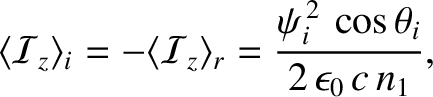Demonstrate that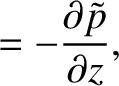whereis the square-root of minus one, and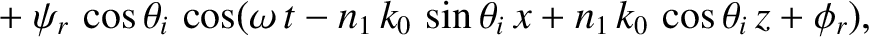. [Hint: You will need to complete the square of the exponent of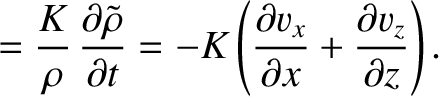, transform the variable of integration, and then make use of the standard result that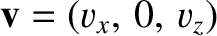.] Hence, show from Euler's theorem,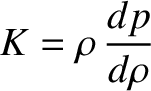, that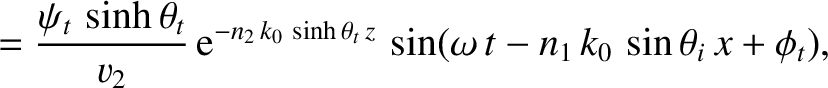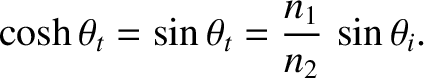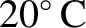3. Demonstrate that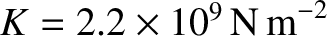4. Verify Equations (728) and (729).
5. Derive Equations (708) and (709) directly from Equation (707) using the results (727)-(729).
6. Verify directly that Equation (746) is a solution of the wave equation, (730), for arbitrary pulse shapes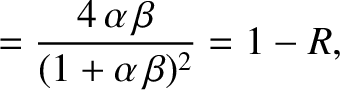and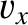.
7. Verify Equation (750).

8. Consider a function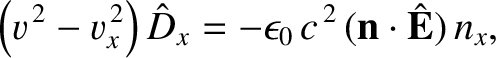that is zero for negative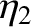, and takes the value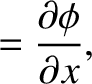for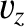. Find its Fourier transforms,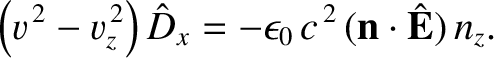and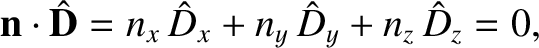, defined in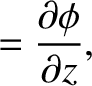[Hint: Use Euler's theorem.]

9. Letbe zero, except in the interval from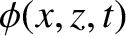to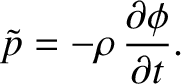. Suppose that in this intervalmakes exactly one sinusoidal oscillation at the angular frequency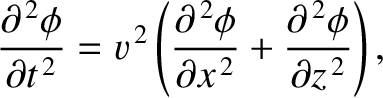, starting and ending with the value zero. Find the previously defined Fourier transformsand.

10. Demonstrate that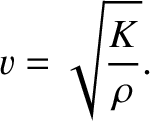where the relation between,, andis as previously defined. This result is known as Parseval's theorem.

11. Suppose thatand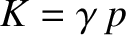are both even functions ofwith the cosine transforms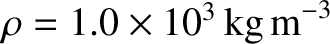and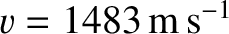, so that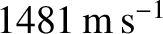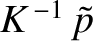Let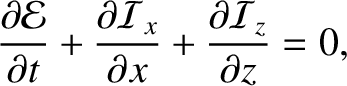, and let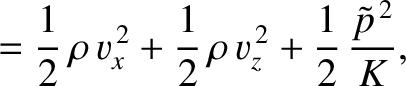be the cosine transform of this even function, so that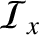Demonstrate that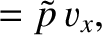This result is known as the convolution theorem, since the above type of integral is known as a convolution integral. Suppose that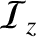. Show that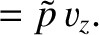12. Demonstrate thatwhereis an arbitrary function, and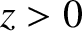a constant unit vector, is a solution of the three-dimensional wave equation, (537). How would you interpret this solution?
13. Demonstrate that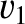whereis an arbitrary function, is a solution of the spherical wave equation, (540). How would you interpret this solution?Next: Dispersive Waves Up: Wave Pulses Previous: Bandwidth
Richard Fitzpatrick 2013-04-08